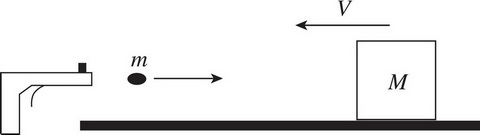# AP Physics 1: Linear Momentum Multiple-Choice Practice Questions

### Test Information11 questions19 minutes

1. An object of mass 2 kg has a linear momentum of magnitude 6 kg · m/s. What is this object's kinetic energy?

2. A ball of mass 0.5 kg, initially at rest, acquires a speed of 4 m/s immediately after being kicked by a force of strength 20 N. For how long did this force act on the ball?

3. A box with a mass of 2 kg accelerates in a straight line from 4 m/s to 8 m/s due to the application of a force whose duration is 0.5 s. Find the average strength of this force.

4. A ball of mass m traveling horizontally with velocity v strikes a massive vertical wall and rebounds back along its original direction with no change in speed. What is the magnitude of the impulse delivered by the wall to the ball?

5. Two objects, one of mass 3 kg moving with a speed of 2 m/s and the other of mass 5 kg and speed 2 m/s, move toward each other and collide head-on. If the collision is perfectly inelastic, find the speed of the objects after the collision.

6. Object 1 moves toward Object 2, whose mass is twice that of Object 1 and which is initially at rest. After their impact, the objects lock together and move with what fraction of Object 1's initial kinetic energy?

7. Two objects move toward each other, collide, and separate. If there was no net external force acting on the objects, but some kinetic energy was lost, then

8. Two frictionless carts (mass = 500 g each) are sitting at rest on a perfectly level table. The teacher taps the release so that one cart pushes off the other. If one of the carts has a speed of 2 m/s, then what is the final momentum of the system (in kg · m/s)?

9. A wooden block of mass M is moving at speed V in a straight line.How fast would the bullet of mass m need to travel to stop the block (assuming that the bullet became embedded inside)?

10. Which of the following best describes a perfectly inelastic collision free of external forces?

11. Object 1 moves with an initial speed of v0 toward Object 2, which has a mass half that of Object 1. If the final speed of both objects after colliding is 0, what must have been the initial speed of Object 2 (assuming no external forces)?